## A student ran out of time on a multiple choice exam and randomly guessed the answers for two problems. Each problem had 5 answer choices – a

Question

A student ran out of time on a multiple choice exam and randomly guessed the answers for two problems. Each problem had 5 answer choices – a, b, c, d, e- and only one correct answer. What is the probability that she answered neither of the problems correctly? Do not round your answer. (If necessary, consult a list of formulas.)​

in progress 0
6 months 2021-07-26T12:53:38+00:00 1 Answers 55 views 0

## Answers ( )

there is a 64% chance that the student got both problems wrong

a 32% chance that they got only 1 correct

and a 4% chance that they got both correct

Step-by-step explanation:

There are 25 total possible combinations of answers, with 8 possible combinations where the student would get 1 answer right, and 1 combination where the student would get both answers correct.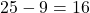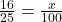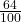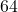%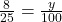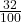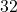%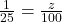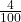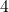%